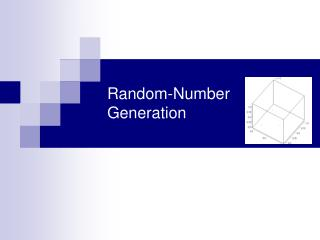Download PresentationRandom-Number Generation# Random-Number Generation - PowerPoint PPT Presentation

Download Presentation##### Random-Number Generation

Download Policy: Content on the Website is provided to you AS IS for your information and personal use and may not be sold / licensed / shared on other websites without getting consent from its author. While downloading, if for some reason you are not able to download a presentation, the publisher may have deleted the file from their server.

- - - - - - - - - - - - - - - - - - - - - - - - - - - E N D - - - - - - - - - - - - - - - - - - - - - - - - - - -
##### Presentation Transcript

1. Random-Number Generation

2. Properties of Random Numbers • Random Number, Ri, must be independently drawn from a uniform distribution with pdf: • Two important statistical properties: • Uniformity • Independence. U(0,1) Figure: pdf for random numbers

3. Generation of Pseudo-Random Numbers • “Pseudo”, because generating numbers using a known method removes the potential for true randomness. • Goal: To produce a sequence of numbers in [0,1] that simulates, or imitates, the ideal properties of random numbers (RN). • Important considerations in RN routines: • Fast • Portable to different computers • Have sufficiently long cycle • Replicable • Closely approximate the ideal statistical properties of uniformity and independence.

4. Linear Congruential Method [Techniques] • To produce a sequence of integers, X1, X2, … between 0 and m-1 by following a recursive relationship: • The selection of the values for a, c, m, and X0 drastically affects the statistical properties and the cycle length. • The random integers are being generated [0,m-1], and to convert the integers to random numbers: The modulus The multiplier The increment

5. Examples[LCM] • Use X0 = 27, a = 17, c = 43, and m = 100. • The Xi and Ri values are: X1 = (17*27+43) mod 100 = 502 mod 100 = 2, R1 = 0.02; X2 = (17*2+43) mod 100 = 77, R2 = 0.77; X3 = (17*77+43) mod 100 = 52, R3 = 0.52; X4 = (17* 52 +43) mod 100 = 27, R3 = 0.27 • “Numerical Recipes in C” advocates the generator a = 1664525, c = 1013904223, and m = 232 • Classical LCG’s can be found on http://random.mat.sbg.ac.at

6. Characteristics of a Good Generator[LCM] • Maximum Density • Such that the values assumed by Ri, i = 1,2,…, leave no large gaps on [0,1] • Problem: Instead of continuous, each Ri is discrete • Solution: a very large integer for modulus m • Approximation appears to be of little consequence • Maximum Period • To achieve maximum density and avoid cycling. • Achieve by: proper choice of a, c, m, and X0. • Most digital computers use a binary representation of numbers • Speed and efficiency are aided by a modulus, m, to be (or close to) a power of 2.

7. A Good LCG Example X=2456356; %seed value for i=1:10000, X=mod(1664525*X+1013904223,2^32); U(i)=X/2^32; end edges=0:0.05:1; M=histc(U,edges); bar(M); hold; figure; hold; for i=1:5000, plot(U(2*i-1),U(2*i)); end

8. Randu (from IBM, early 1960’s) X=1; %seed value for i=1:10000, X=mod(65539*X+57,2^31); U(i)=X/2^31; end edges=0:0.05:1; M=histc(U,edges); bar(M); hold; figure; hold; for i=1:3333, plot3(U(3*i-2),U(3*i-1),U(3*i)); end Marsaglia Effect (1968)

9. Random-Numbers Streams[Techniques] • The seed for a linear congruential random-number generator: • Is the integer value X0 that initializes the random-number sequence. • Any value in the sequence can be used to “seed” the generator. • A random-number stream: • Refers to a starting seed taken from the sequence X0, X1, …, XP. • If the streams are b values apart, then stream i could defined by starting seed: • Older generators: b = 105; Newer generators: b = 1037. • A single random-number generator with k streams can act like k distinct virtual random-number generators • To compare two or more alternative systems. • Advantageous to dedicate portions of the pseudo-random number sequence to the same purpose in each of the simulated systems.